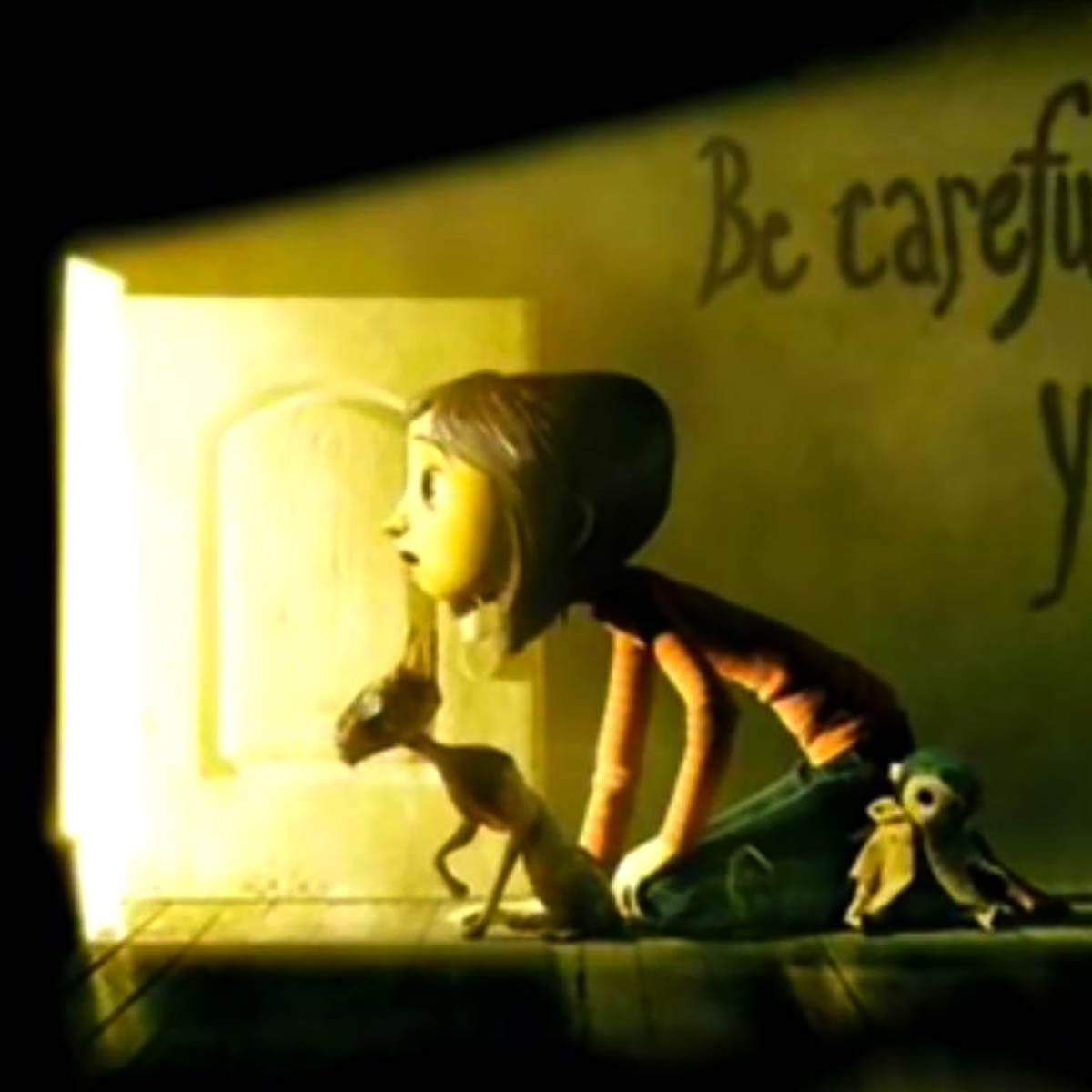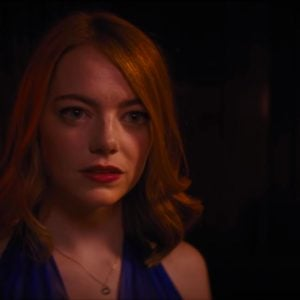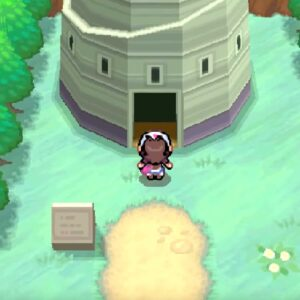# Coraline Exploration (Coraline)

27 August 2020
4
• ##### TYPE
0
(0)

o P o i i o O o [OH] [PJ] P O o o i Y i Y T Y E D P O S P [iP] D P Y Y P O o i Y P O o o i Y i i i i T T T i O o P Y O o O i Y T i i i T T T i O o P H J D S D J D [DG] g G H G g G J D P D g G H G g S g H D D W i T I D S a P O I i Y Y P [oP] P [oP] Y [oP] [iO] [oP] P [oP] Y [oP] [YoP] Y [oP] [YiO] [oD] [YD] o i [JY] H [JY] J P o [Hi] h [Hi] o O o O [JP] P O o o i Y [iJ] i i L l J [YH] [TJ] Y J o i Y [PY] Y O i Y o i P T O o i o h g D J H [hP] D D P Y o i Y o i Y P [YO] o [io] Y o Y [Yi] Y Y Y

Rate This Music Sheet:

Average rating 0 / 5. Vote count: 0

No votes so far! Be the first to rate this music sheet.

Thank you for rating this song!

If you have any specific feedback about how to improve this music sheet, please submit this in the box below.

Coraline Exploration (Coraline) is a song by Bruno Coulais. Use your computer keyboard to play Coraline Exploration (Coraline) music sheet on Virtual Piano. This is an Easy song and requires practice. The song Coraline Exploration (Coraline) is classified in the genre of Songs From Movies on Virtual Piano. You can also find other similar songs using Animation, Universal Pictures.

## Related

•OSDfDSp pSDfDSO OSDfDSp GfDfDSO j HGHGfSD H GfGfDS j HGHGfSDH LkjkjHG ZLkjHGf SDSDf SDSDf SDSDfSHH QWe TYuIOp TYuIOp SDfSDfGHjkL GHjkLZxCV LZxCVbn
Level: 3
Easy

#### Justin Hurwitz

•Opap O u T|u T T u Op O I Y Yu u u| TYu u u| Opap O u T|u T T u Op O I a Yu u u| SDf f f| u T TYua p O a O u Iu|y T Y ut Y u I I u IO|u T TYua p O f D a O au|y T|u Yu I u| Opap O u T|u T T u Op O I Y Yu u u| TYu u u| Opap O u T|u T T u Op O I a Yu u u| SDf f f| u T TYua p O a O u Iu|y T Y ut Y u I I u IO|u T TYua p O f D a O au|y T|u Yu I u| Op

Level: 2
Length: 01:04
Easy

#### Junichi Masuda

•q [et] [et] 8 [et] [et] 9 [et] [et] 8 9 g [0j] g [qf] [et] [et] 8 [et] g [etj] f [9d] [et] d [etg] d [8s] [et] [et] d [7j] [9qwh] [9qw] d [7j] [9qwh] f [9qwg] [7d] [9qw] [9qw] 7 [9qw] g [9qwh] g [0f] [wt] [wt] 9 [wt] f [wth] f [8s] [0w] s [0wf] s [^P] [0w] P [0ws] P q [etp] [etP] [qs] [et] d [etf] 8 [0ws] [0wd] [80wf] g j g [qf] [et] [et] 8 [et] g [etj] f [9d] [et] d [etg] d [8s] [et] [et] d [7j] [9qwh] [9qw] d [7j] [9qwh] f [9qwg] [7d] [9qw] [9qw] 7 [9qw] g [9qwh] g [0f] [wt] [wt] 9 [wt] f [wth] f [8s] [0w] s [0wf] s [^P] [0w] P [0ws] P q [etp] [etP] [qs] [et] d [etf] 8 [0ws] [0wd] [80wf] g j g [qf] [et] [et] 8 [et] g [etj] f [9d] [et] g [etj] z [8x] [et] [et] z [7c] [9qwx] [9qw] z [7j] [9qwk] [9qwz] z x [7c] [9qw] [9qw] c x [7z] [9qw] c [9qwv] c [0wtx] [9wt] x v x [80wl] l x l [0w^J] l z x v b [8B] m B m B m B m [0wB] m B m B m B m [0wB] m B m [5B] [0w] [0w] [8B] [0wb] [0w] h [5z] [0wj] [0wh] [4j] [qe] [qe] [8g] [qe] [qe] [4j] [qeh] [qe] g [2j] [qrG] [qrd] [7a] [qrL] z Z x c C v V b B [9B] b [qrV] v C c x Z z L l a [0wtf] h d f P a [etus] l z x c v b c [qx] [et] 8 [et] c b x [9z] [et] z c b [8m] [et] c [7b] [wrv] z [5b] [wrv] x c [7z] [wr] 9 [wr] c v c [0x] [wt] 9 [wt] x v x [8l] [0w] l x l [^P] [qet] p j P J s l d z f x [80w] s l d z f x g j g [qf] [et] [et] 8 [et] [et] 9 [et] [et] [et] 8 [et] g j g f i u i g j g [qetf] [0et] g j f [qetd] d g d [(ets] a [9wrj] h d [9wj] h g [9wrd] [9w] g h g [0wtf] [9wt] f h f [80ws] s f s [0w^P] [qet] p P s d f [0wt] s d f [pg] [pf] [pg] [pf] c b c [pgx] [pf] c b x [pgz] z c z [Ypsl] z [ryob] v z [eyob] v c [wryz] [qry] c v c [sfx] s x v x [Posl] l x l [oPJ] [tip] j J l z x [wtu] l z x c b c [qetm] [qetu] [qet] [qetu] [8qeti]
Level: 6
Length: 04:11
Intermediate